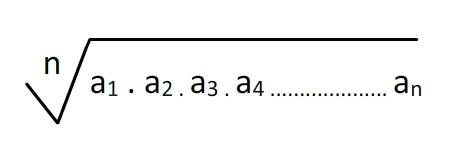﻿ Geometric Mean Calculator | Calculate geometric mean online

# Geometric Mean Calculator

Geometric mean calculator is an online tool to calculate the geometric mean of a series containing n observations, it is the nth root of the product of the values. GM is used in studies like bacterial growth, cell division, etc.

#### Formula to Calculate Geometric Mean## What is Geometric Mean

Geometric means are a type of average, or measure of central tendency in a distribution of data points, in the same group as the median, mode, or arithmetic mean. Whereas the arithmetic mean is calculated by summing a series of data points and then dividing that sum by the number of data points, the geometric mean multiplies a series of data points, and then uses the n number of data points to find the nth root of that product. Mathematically, the geometric mean adds depth and stability to the mean.

We can easily visualize the geometric mean when applying it to its counterpart, the geometric series of numbers, where each number increases from the previous number according to the same proportion. The geometric mean will lie in the direct center of the values, whereas the arithmetic mean would have been pulled towards the higher values, and thus not truly represent the center of the data

### When should I use the geometric mean instead of the arithmetic mean?

There are no hard rules for which mean you should use. Different types of averages can be used to express slightly different concepts: the center of the data, the values most often seen, and/or the typical “expected” values may or may not all be conveyed by the same measure. Data is rarely perfect, and you may need to look at several different types of averages to decide what works best for what you are trying to communicate with your data. But in general, geometric means are preferable when looking at skewed data, scaled data, or when averaging ratios. Some common applications include:

• Population growth
• Compounding interest
• Bioassays